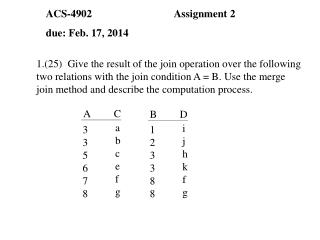# ACS-4902 Assignment 2 due: Feb. 17, 2014 - PowerPoint PPT PresentationDownload PresentationACS-4902 Assignment 2 due: Feb. 17, 2014

Presentation Description
Download Presentation## ACS-4902 Assignment 2 due: Feb. 17, 2014

- - - - - - - - - - - - - - - - - - - - - - - - - - - E N D - - - - - - - - - - - - - - - - - - - - - - - - - - -
##### Presentation Transcript

1. i j h k f g 1 2 3 3 8 8 ACS-4902 Assignment 2 due: Feb. 17, 2014 1.(25) Give the result of the join operation over the following two relations with the join condition A = B. Use the merge join method and describe the computation process. A C B D a b c e f g 3 3 5 6 7 8

2. PREREQUISITE SECTION CourseNum=CourseNum CourseNum=CourseNum ACS-4902 Assignment 2 due: Feb. 17, 2014 2.(25) Generate an optimal query tree for the following query (see Fig. 1.2 for relation schemas): Name, StuNumber((Dept=‘BC’(COURSE) GRADE_REPORT) STUDENT) SEcIdentifier=SecIdentifier StuNumber=StuNumber

3. ACS-4902 Assignment 2 due: Feb. 17, 2014 3.(25) Assume that X = 90, Y = 100, N = 12, M = 8. Show the the results by executing the following interleaved transactions. Are the results correct? Explain your results. TimeTransaction1Transaction2 1 READ(X) 2 X:=X-N 3 READ(X) 4 X:=X+M 5 WRITE(X) 6 READ(Y) 7 WRITE(X) 8 Y:=Y+N 9 WRITE(Y)

4. ACS-4902 Assignment 2 due: Feb. 17, 2014 4.(25) Given the following schedules. Show which is recoverable, cascadeless or strict. Explain why. i) R1(X), R2(X), R1(Y), R2(Z), W1(X), R1(Z), W2(Z), C2, W1(Y), C1. ii) R1(X), W1(X), R2(Z), R2(Y), R2(X), W2(Y), C2, A1. iii) R1(X), W1(X), R1(Y), R2(Y), W2(Y), W1(Y), C1, R2(X), W2(X), C2.